# Values Drop Zone

The Values drop zone drives the quantitative data in the query. As such it's arguably the most important drop zone, since almost every visualization needs values or metrics to sow useful results.

The Values zone is where values (measures) are added to the query. The behavior of the Values zone differs according to the visualization type selected - including sub-drop zone options that drive the handling of the measure chips in the context of the relevant visualization.

### Grids

When working with grids, the Values zone works in conjunction with the Columns and Rows zones.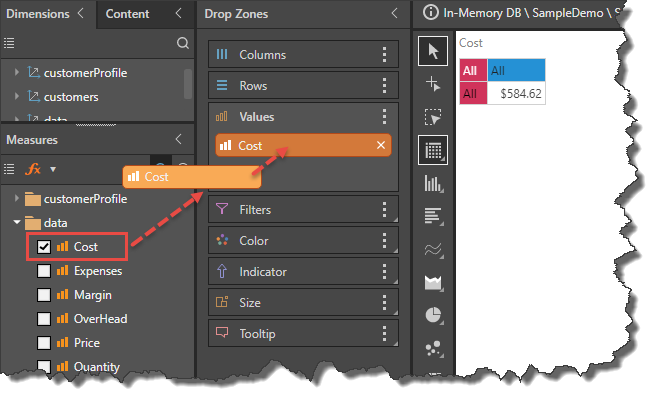A measure may be added to the Values zone by:

• Dragging and dropping it into the Values zone.
• Selecting the measure's check box.
• Double clicking on the measure.
• Right clicking on the measure and selecting Values from the context menu (see image below).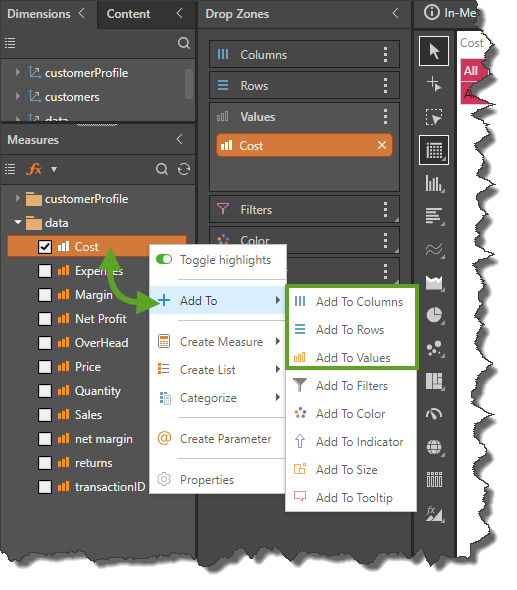### Values Chip

An orange Values chip is automatically generated when a measure is added to Columns or Rows, or when more than one measure is added to the query. The Measure chips will remain in the Values zone, while the Values chip can be placed wherever required. The placement of Values chip in the drop zones then drives the location of the measure(s) in the visualization.

#### Multiple Measure Handling

When working with grids, if more than one measure is added to the query, a Values chip will be generated and added to Columns initially. The Values chip determines the location of the measures in the grid.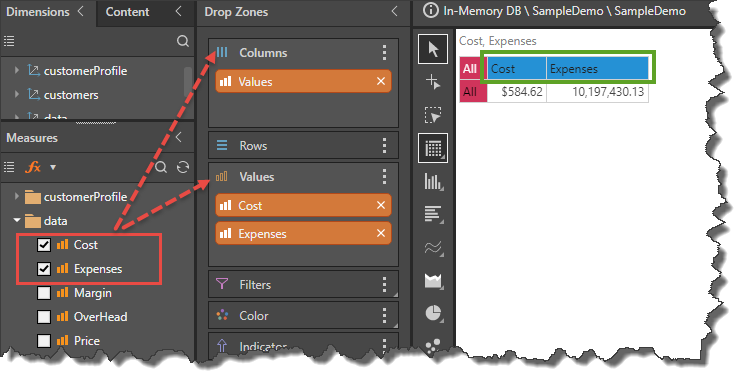This can then be moved to another drop zone (like rows) to position the multiple measures differently within the visualization. In this example, the Values chip was moved from Columns (image above) to Rows (image below):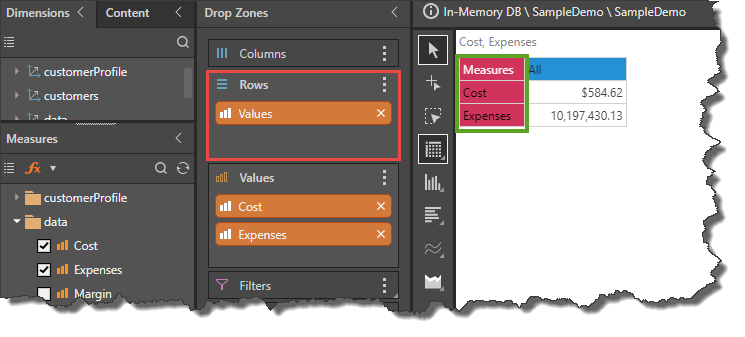The Values chip can also be moved to the Filters zone to generate a measure slicer from which the required measure can be selected. This doesn't choose a classic "slice" (or data filter) of the data model to show; instead it switches out the measure being used in the visualization.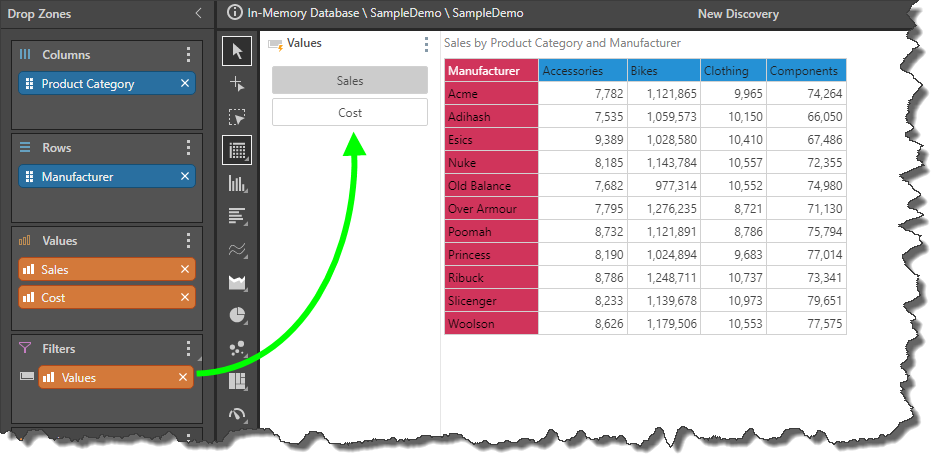## Single Metric Charts

When working with most charts at least 1 measure must be added to the Values drop zone. For other chart visualizations (like scatter and map charts), two or more measures may be needed.

In the example below the measure drives the column chart's values and is effectively shown in the height of the columns and the chart's y-axis. So depending on the chart type, the measure graphically drives the size, height, angle or length of the chart's data points.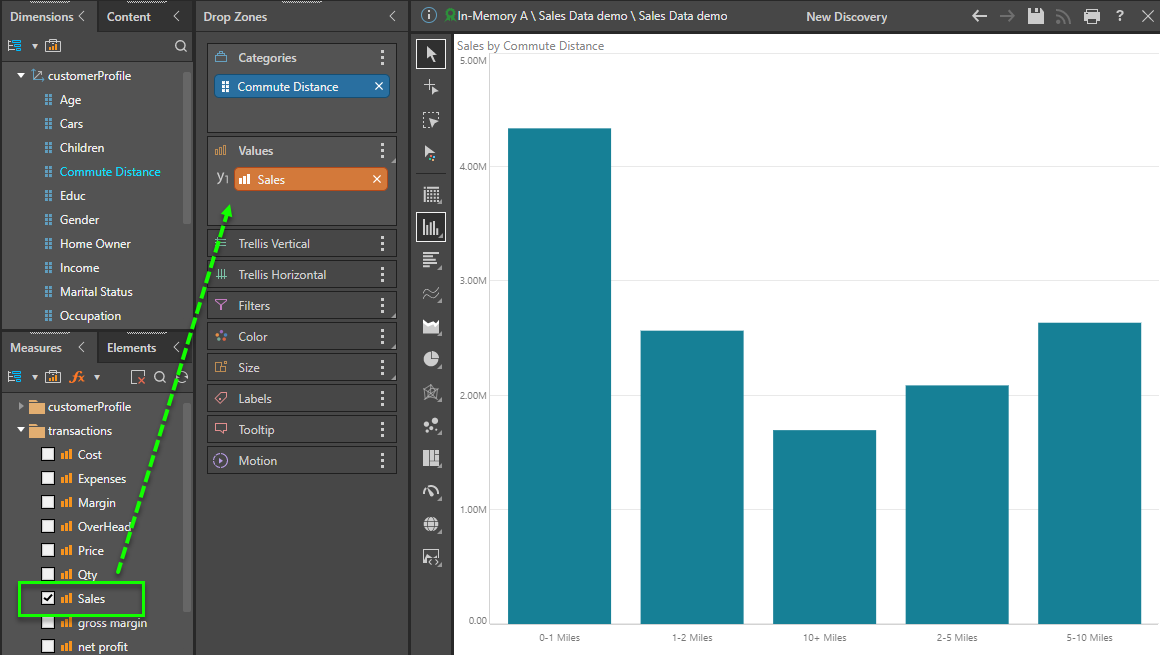### Values Chip

If the measure chip is moved to one of the Trellis zones, or to the Filters zone, a Values chip is automatically generated. By default, the Values chip is also generated if more than one measure is added to the Values zone (where the base chart visual only needs 1 measure). If the second (and subsequent) measure chips are instead added as "primary axis" or "secondary axis" chips, the Values chip is not generated. (See below for more on multi-measure handling). The given measures will remain in the Values zone, while the Values chip can be placed wherever required. The placement of the Values chip drives the location of the measures in the chart.

#### Multiple Measure Handling

When using multiple measures in many charts, there are a variety of choices and options that can be employed to achieve different effects and effectively, different chart types and / or reports. Overall, there are 4 different effects: Standard Trellising (default), Primary Axis, Secondary Axis and Differentiated Trellising. The last 3 choices are mainly for Cartesian charts (column, bar, line, area, point etc).

##### Standard Trellising

By default, if more than one measure is added to Values, the Values chip is generated and added to Trellis Vertical, resulting in multiple plotted charts. In the example below, 2 column charts are created for Cost and Expenses, with the charts being vertically trellised (so the Values chip is placed in the Vertical Trellis drop zone). The Values chip may be moved to Trellis Horizontal or Filters to change the effect and report.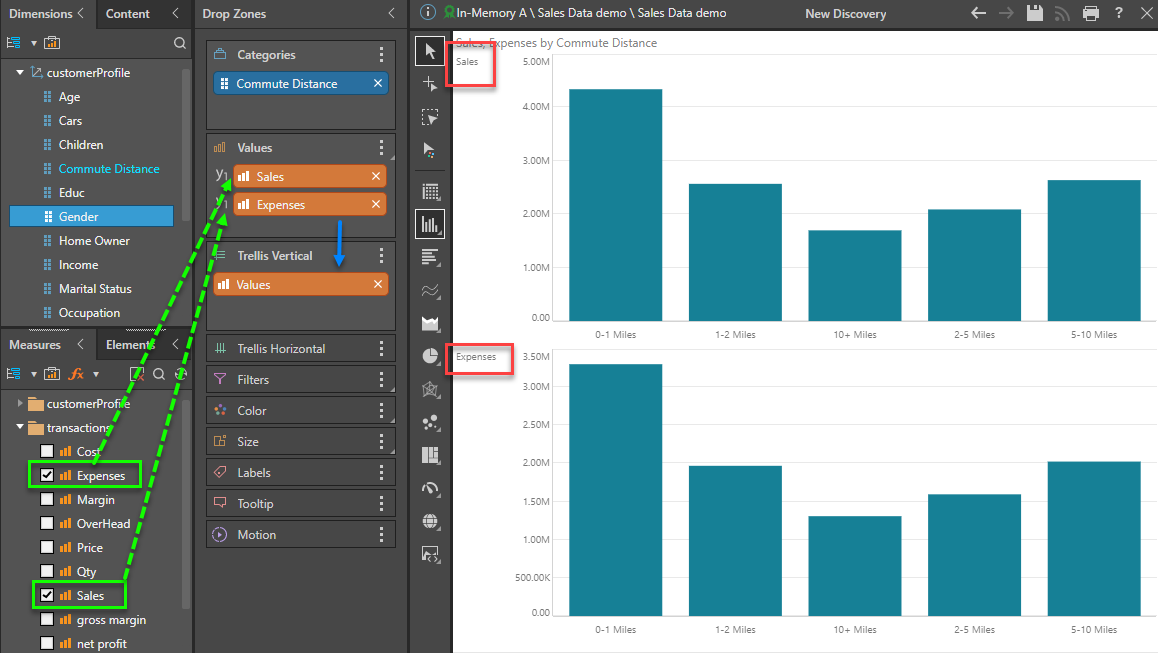##### Primary Axis - Multi-Measures

When working with Cartesian and Segment charts it's possible to create multi-measure charts that use the SAME y-axis. This includes standard charts, stacked charts, or multi-measure segment charts.

In the example below, the Expenses measure is dragged to the Value drop zone and then using the sub-drop zone of "Primary Axis."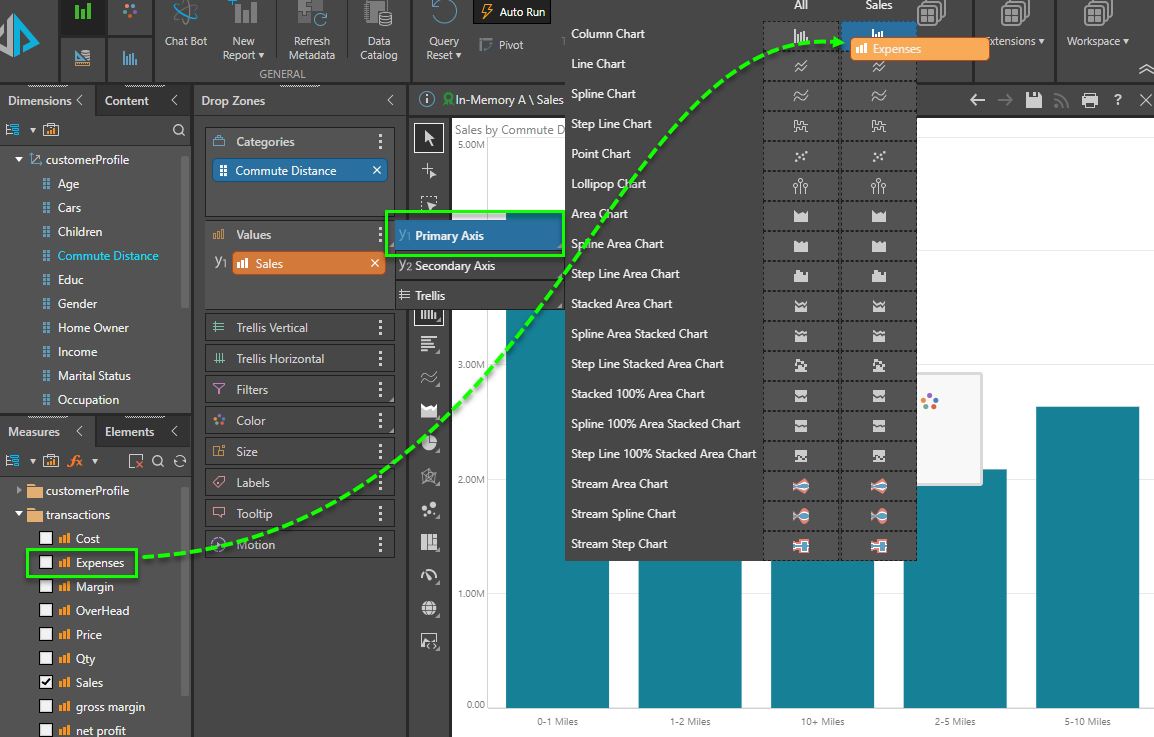In this scenario, the resulting chart (below) shows both metric values shown in the same plot area, sharing the same common y-axis. Note there is no Value chip and no trellising. The measures are also auto-colorized so each one has its own distinct color.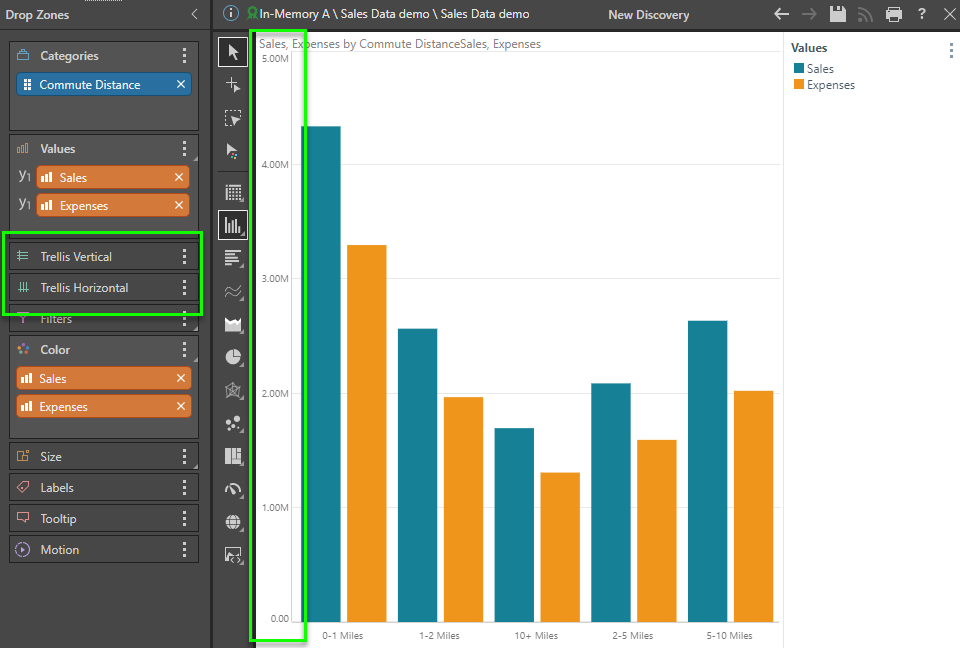##### Secondary Axis - Combo Charts

When working with Cartesian charts it's possible to create multi-measure charts that use the different y-axes in the same plot area. Generally referred to as "combo charts", one measure is typically -plotted on the primary y-axis (on the left) and the second measure is plotted on the secondary y-axis on the right. They are also typically different chart types (but do not have to be).

In the example below, the Margin measure is dragged to the Value drop zone and then using the sub-drop zone of "Secondary Axis."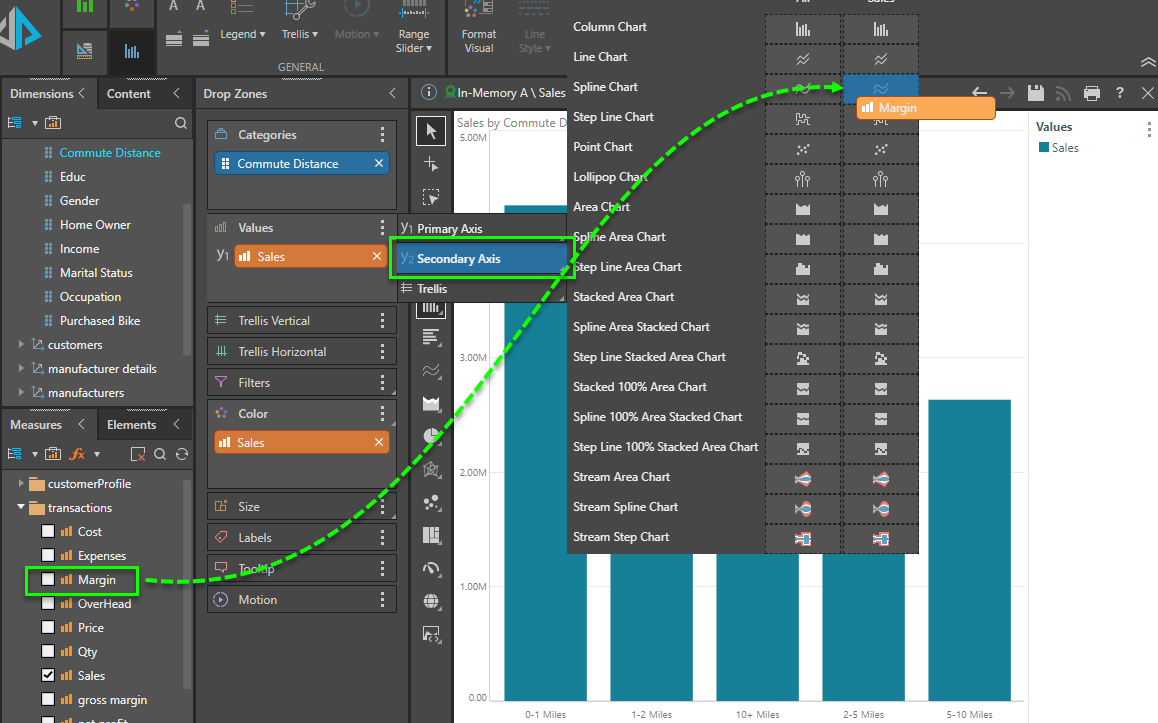In this scenario, the resulting chart (below) shows both metric values shown in the same plot area, using 2 different y-axes. Note there is no Value chip and no trellising. The measures are also auto-colorized so each one has its own distinct color.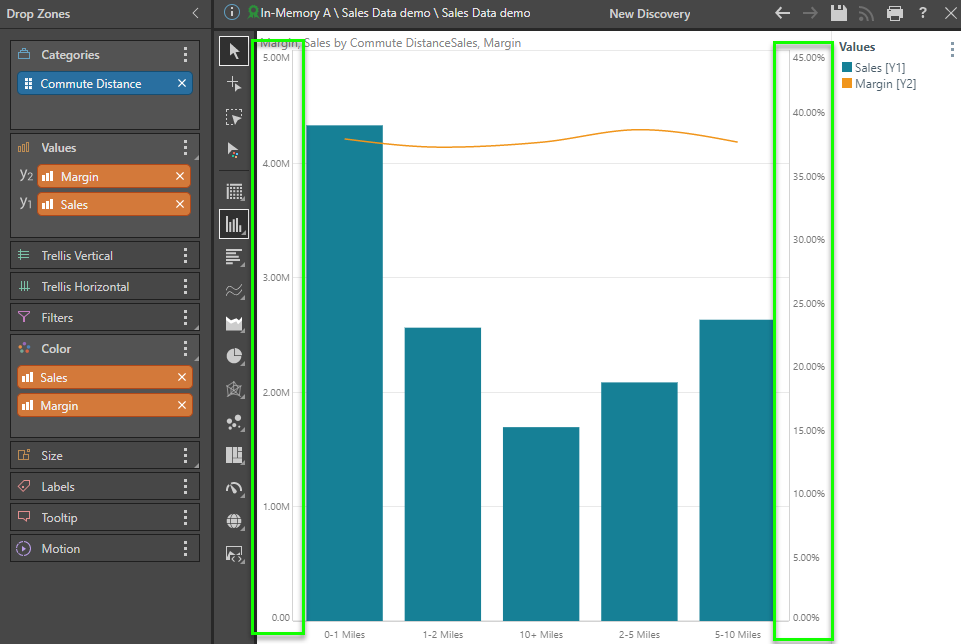##### Differentiated Trellising

When working with Cartesian charts it's possible to create multi-measure charts that use the different plot areas, different y-axes AND different chart styles. Generally referred to as "differentiated trellised" charts they are similar to the standard variety (explained above) - but allow each plotted chart to have its own chart type.

In the example below, the Expenses (the then Margin) metrics are dragged to the Value drop zone and then using the sub-drop zone of "Trellis." The measures are also auto-colorized so each one has its own distinct color.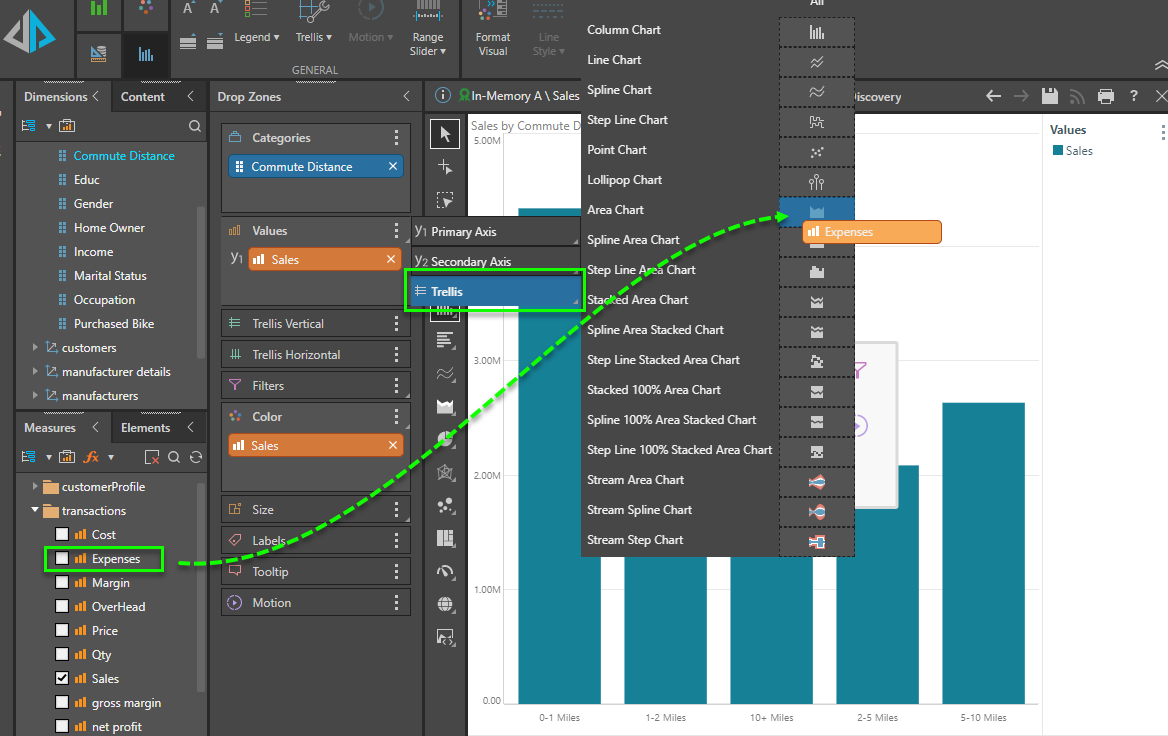In this scenario, the resulting chart (below) shows all 3 metric values with their own plot areas, using 3 different and independent y-axes and 3 different chart types (column, area and spline). Note there is a Value chip in trellising.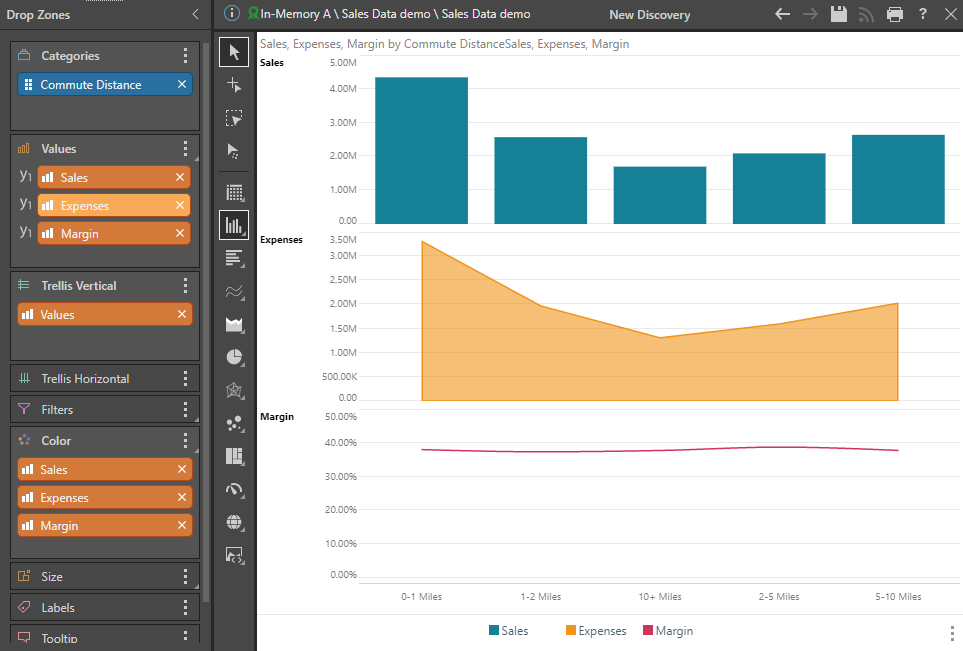## Multi-Metric Charts

Multi-metric charts are charts that require a minimum of 2 or more measures. Unlke single metric charts, that require a minimum of a single measure and can then have additive metrics added to create variations (as described above), multi-metric charts are by definition dysfunctional without the extra measures. The processes described above for single charts applies to multi-metric charts as well, but there are subtle differences.

In the example below, the scatter plot requires an x and y measure to be operational.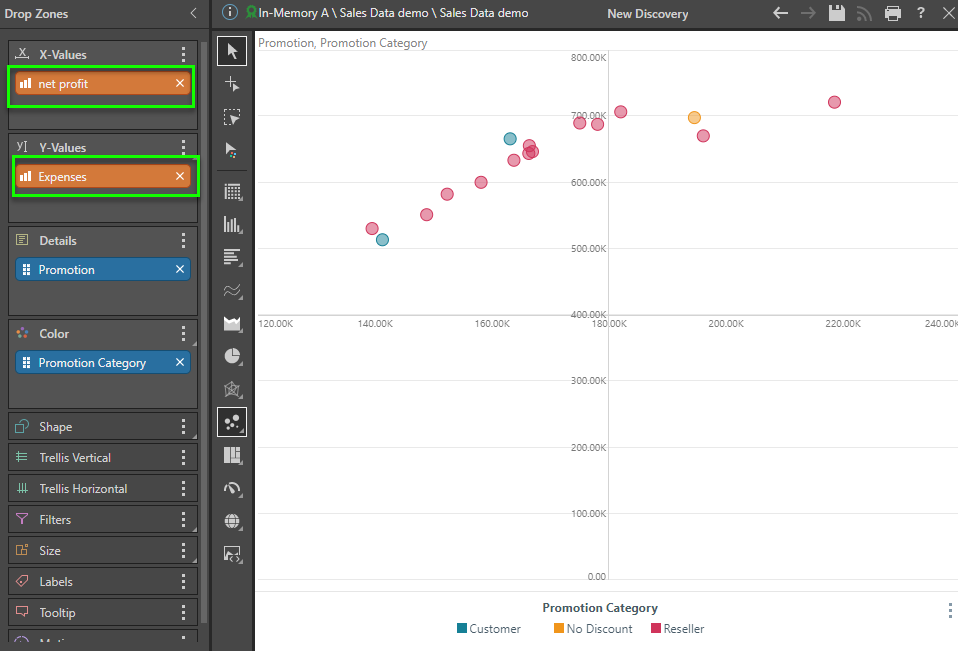Adding another measure to the y-axis, creates the standard trellising effect described above. as can be seen in the chart below. Here, 2 different scatters charts are drawn, with their own pot areas - one for net profit and expenses; and another for net profit and cost.

##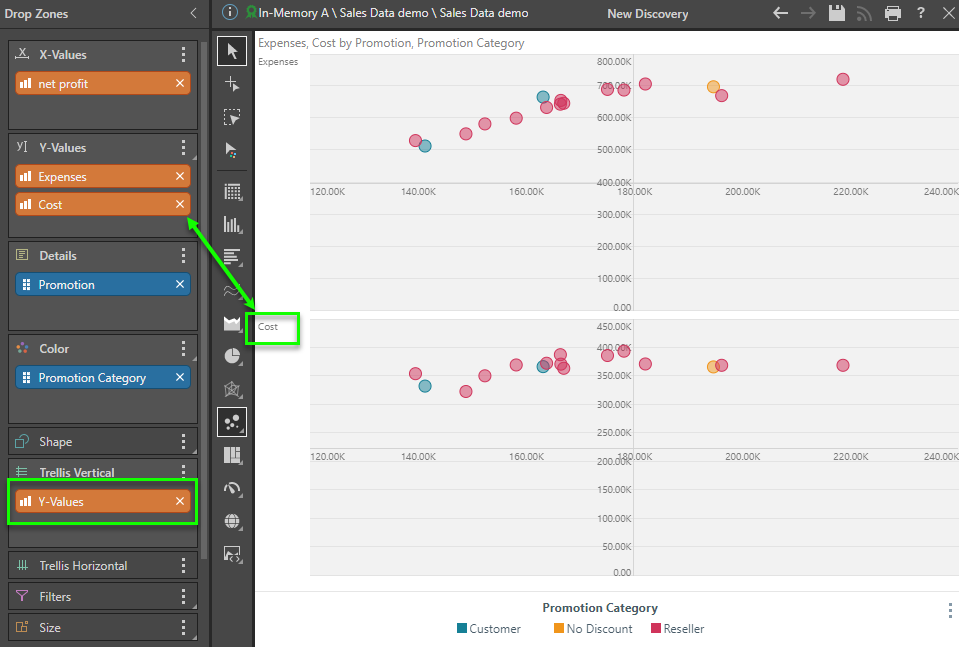Connecting the Values Drop Zone to Colors and Shapes

Usually, when using multiple measures in charts (and sometimes in grids), the different metrics need to be "colorized" into different colors. This concept is an extension to the above concepts where a user might use multiple measures in the same plot area and needs to delineate the graphic with colors. The same may apply to "shapes" or the icons of data points used in charts like point charts, line charts, scatter plots or bubble charts.

To accelerate the setup of these capabilities, users can access 2 quick macro functions:

• Colorize - which automatically sets each measure with its own color. Click here for more details.
• Shapify - which automatically sets each measure with its own data point shape. Click here for more details.